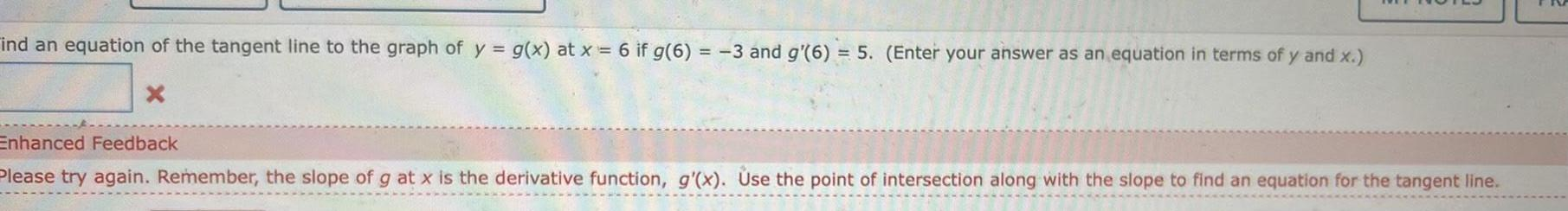Question:

# ind an equation of the tangent line to the graph of y g x at

Last updated: 9/18/2023ind an equation of the tangent line to the graph of y g x at x 6 if g 6 3 and g 6 5 Enter your answer as an equation in terms of y and x X Enhanced Feedback Please try again Remember the slope of g at x is the derivative function g x Use the point of intersection along with the slope to find an equation for the tangent line